# BL-101 – Coriolis Gas Flow Rate

## Description

This block calculates the uncertainty in gas flow rate for a Coriolis meter where mass flow rate, standard volume flow rate and energy flow rate uncertainties are added up to get the expanded uncertainty.

## Options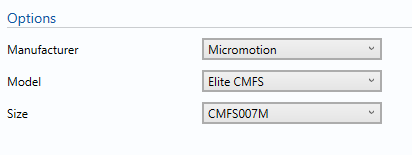### Manufacturer

This option is used to select the manufacturer of the Coriolis meter.

### Model

This option is used to select the model of the Coriolis meter.

### Size

This option is used to select the size of the Coriolis meter.

## Inputs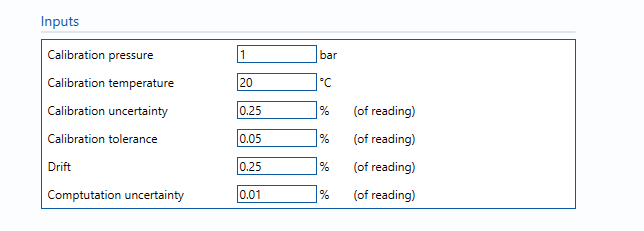Calibration pressure – The pressure at which the Coriolis was calibrated.

Calibration temperature – The temperature at which the Coriolis was calibrated.

Calibration uncertainty – The uncertainty of the reference device used for calibration of the Coriolis meter.

Calibration tolerance – The tolerance criteria used for calibration of the Coriolis meter.

Drift – Allowance for drift. Normally left at zero, unless evidence is present to suggest a higher value.

Computation uncertainty – Uncertainty due to computational errors in flow computer. Negligible term included for completeness – assume 0.01%.

## Calculations

The gas flow rates (mass, standard volume & energy) are calculated.

The units selected for parameters on the Input/Output tab will be applied globally throughout the uncertainty module in use. They will be applied to values used in the uncertainty budget. Inputs that are local to this block can have their units altered within the block.

### Mass flow rate

The uncertainty calculation for the mass flow rate is detailed in the first uncertainty budget table giving a break down of how the overall uncertainty is calculated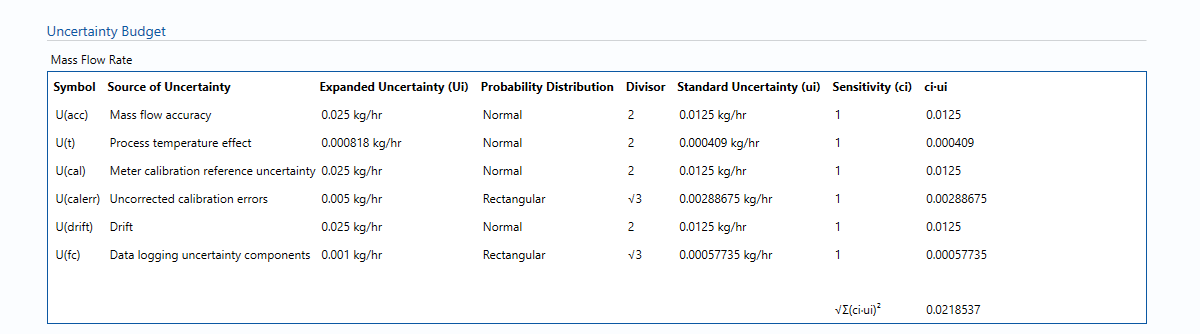The values input into the uncertainty budget are taken from the uncertainty components calculated in this block from the calculation inputs. These values are taken in as the expanded uncertainties and are divided by a coverage factor to gain the standard uncertainty.

The coverage factor is determined by the probability distribution that best suits that uncertainty component. The standard uncertainty is then multiplied by the sensitivity value then squared. This is done for each component that contributes to the overall uncertainty in mass flow rate. The Standard Uncertainty in mass flow rate is the square root of the sum of each component variance as shown in the following equation: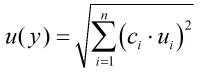### Standard Volume flow rate

The uncertainty calculation for the Standard Volume flow rate is detailed in the uncertainty budget table giving a break down of how the overall uncertainty is calculated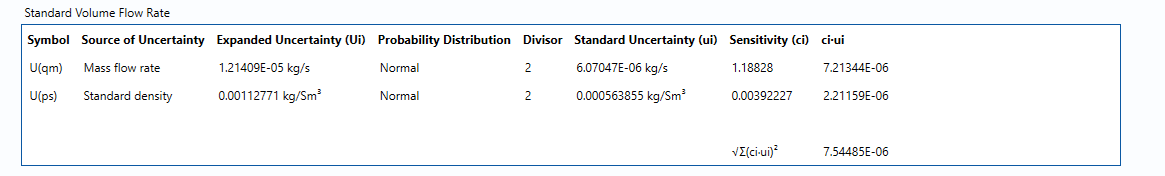The values input into the uncertainty budget are taken from the uncertainty components calculated in this block from the calculation inputs. These values are taken in as the expanded uncertainties and are divided by a coverage factor to gain the standard uncertainty.

The coverage factor is determined by the probability distribution that best suits that uncertainty component. The standard uncertainty is then multiplied by the sensitivity value then squared. This is done for each component that contributes to the overall uncertainty in the standard volume flow rate. The Standard Uncertainty in standard volume flow rate is the square root of the sum of each component variance as shown in the following equation:### Energy flow rate

The uncertainty calculation for the Energy flow rate is detailed in the uncertainty budget table giving a break down of how the overall uncertainty is calculated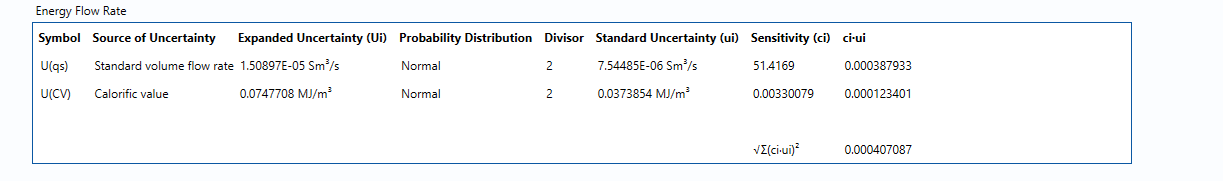The values input into the uncertainty budget are taken from the uncertainty components calculated in this block from the calculation inputs. These values are taken in as the expanded uncertainties and are divided by a coverage factor to gain the standard uncertainty.

The coverage factor is determined by the probability distribution that best suits that uncertainty component. The standard uncertainty is then multiplied by the sensitivity value then squared. This is done for each component that contributes to the overall uncertainty in the energy flow rate. The Standard Uncertainty in energy flow rate is the square root of the sum of each component variance as shown in the following equation:### Calculated Uncertainty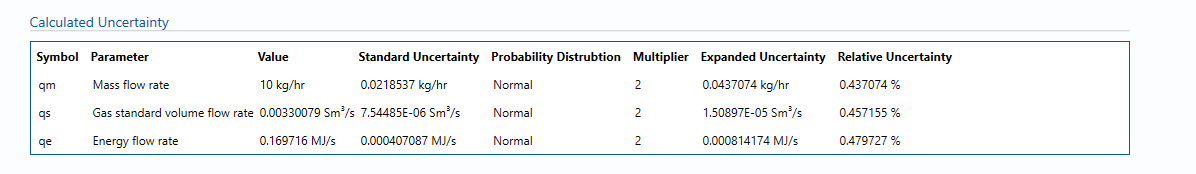The Expanded Uncertainty is the Standard Uncertainty multiplied by the coverage factor (k). The coverage factor is defaulted to k = 2 (equivalent to a confidence level of approximately 95%).

The Relative Uncertainty is the Expanded Uncertainty divided by the mass flow rate or gas standard volume flow rate or energy flowrate depending on the parameter.

### Standards

ISO 5168:2005 – Measurement of fluid flow – Procedures for the evaluation of uncertainties

ISO Guide 98-3 – Uncertainty of measurement – Part 3: Guide to the expression of uncertainty in measurement (GUM:1995)

### Uncertainty Blocks

Pressure

Temperature

Calculated Gas Density

Calculated gas Standard Density

Calorific Value

Back to Uncertainty Modules